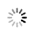# Java 8流解析为整数

5月前 ⋅ 58 阅读

### ChatGPT的回答仅作参考：

```要将Java 8流解析为整数，可以使用`mapToInt()`方法将流中的元素映射为整数流，然后使用`sum()`方法计算整数流的总和。

```java
import java.util.Arrays;
import java.util.List;

public class Main {
public static void main(String[] args) {
List<String> numbers = Arrays.asList("1", "2", "3", "4", "5");

int sum = numbers.stream()
.mapToInt(Integer::parseInt)
.sum();

System.out.println("Sum: " + sum);
}
}
```

```
Sum: 15
``````

### 相关推荐